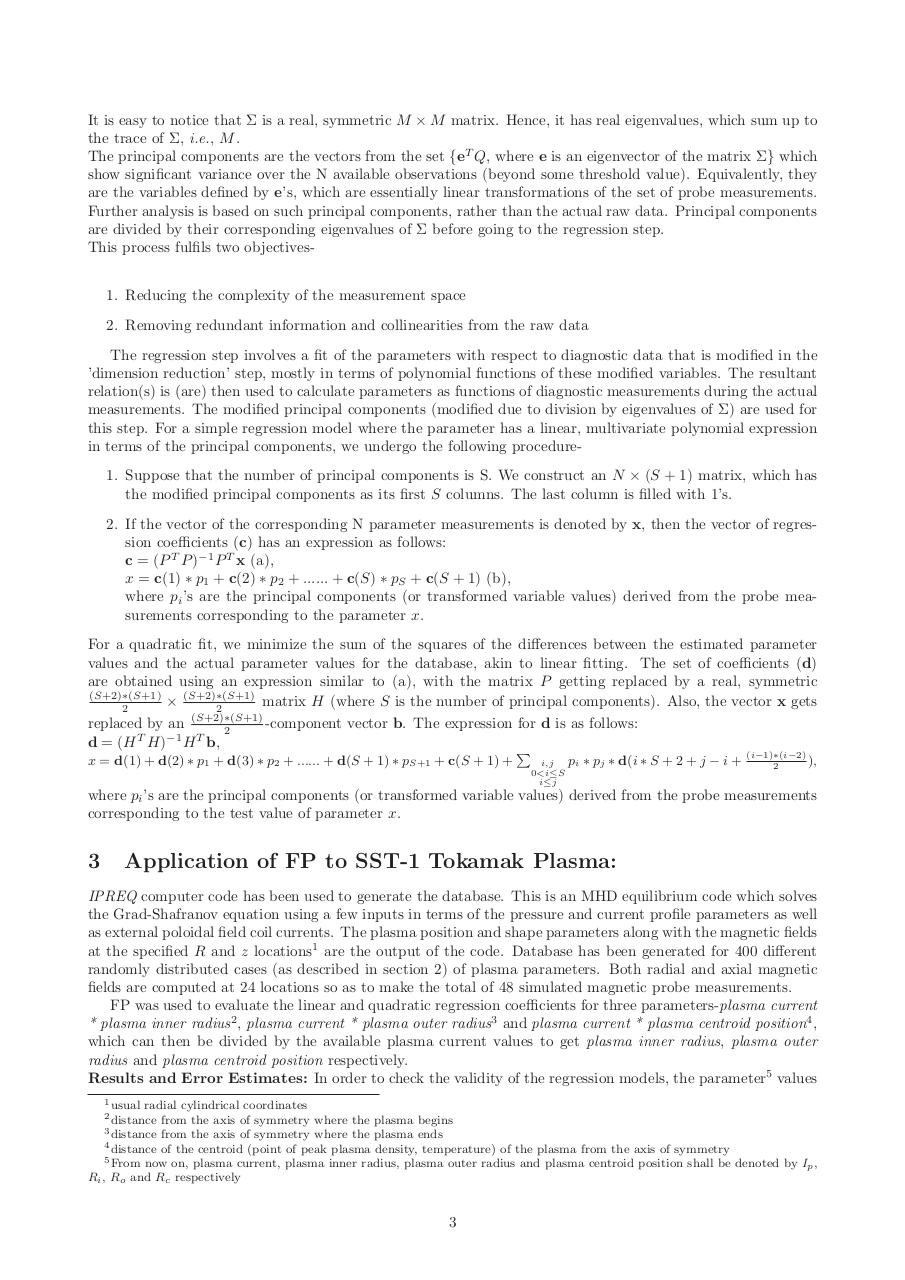# report final.pdfPage 1 2 3 4 5 6 7 8 9 10 11 12

#### Text preview

It is easy to notice that Σ is a real, symmetric M × M matrix. Hence, it has real eigenvalues, which sum up to
the trace of Σ, i.e., M .
The principal components are the vectors from the set {eT Q, where e is an eigenvector of the matrix Σ} which
show significant variance over the N available observations (beyond some threshold value). Equivalently, they
are the variables defined by e’s, which are essentially linear transformations of the set of probe measurements.
Further analysis is based on such principal components, rather than the actual raw data. Principal components
are divided by their corresponding eigenvalues of Σ before going to the regression step.
This process fulfils two objectives1. Reducing the complexity of the measurement space
2. Removing redundant information and collinearities from the raw data
The regression step involves a fit of the parameters with respect to diagnostic data that is modified in the
’dimension reduction’ step, mostly in terms of polynomial functions of these modified variables. The resultant
relation(s) is (are) then used to calculate parameters as functions of diagnostic measurements during the actual
measurements. The modified principal components (modified due to division by eigenvalues of Σ) are used for
this step. For a simple regression model where the parameter has a linear, multivariate polynomial expression
in terms of the principal components, we undergo the following procedure1. Suppose that the number of principal components is S. We construct an N × (S + 1) matrix, which has
the modified principal components as its first S columns. The last column is filled with 1’s.
2. If the vector of the corresponding N parameter measurements is denoted by x, then the vector of regression coefficients (c) has an expression as follows:
c = (P T P )−1 P T x (a),
x = c(1) ∗ p1 + c(2) ∗ p2 + ...... + c(S) ∗ pS + c(S + 1) (b),
where pi ’s are the principal components (or transformed variable values) derived from the probe measurements corresponding to the parameter x.
For a quadratic fit, we minimize the sum of the squares of the differences between the estimated parameter
values and the actual parameter values for the database, akin to linear fitting. The set of coefficients (d)
are obtained using an expression similar to (a), with the matrix P getting replaced by a real, symmetric
(S+2)∗(S+1)
× (S+2)∗(S+1)
matrix H (where S is the number of principal components). Also, the vector x gets
2
2
replaced by an (S+2)∗(S+1)
-component vector b. The expression for d is as follows:
2
d = (H T H)−1 H T b,
x = d(1) + d(2) ∗ p1 + d(3) ∗ p2 + ...... + d(S + 1) ∗ pS+1 + c(S + 1) +

P

i,j
0&lt;i≤S
i≤j

pi ∗ pj ∗ d(i ∗ S + 2 + j − i +

(i−1)∗(i−2)
),
2

where pi ’s are the principal components (or transformed variable values) derived from the probe measurements
corresponding to the test value of parameter x.

3

Application of FP to SST-1 Tokamak Plasma:

IPREQ computer code has been used to generate the database. This is an MHD equilibrium code which solves
the Grad-Shafranov equation using a few inputs in terms of the pressure and current profile parameters as well
as external poloidal field coil currents. The plasma position and shape parameters along with the magnetic fields
at the specified R and z locations1 are the output of the code. Database has been generated for 400 different
randomly distributed cases (as described in section 2) of plasma parameters. Both radial and axial magnetic
fields are computed at 24 locations so as to make the total of 48 simulated magnetic probe measurements.
FP was used to evaluate the linear and quadratic regression coefficients for three parameters-plasma current
* plasma inner radius2 , plasma current * plasma outer radius3 and plasma current * plasma centroid position4 ,
which can then be divided by the available plasma current values to get plasma inner radius, plasma outer
radius and plasma centroid position respectively.
Results and Error Estimates: In order to check the validity of the regression models, the parameter5 values
1 usual